Today we will work with data from Kaggle on NFL players. You should have a copy of the data file already.

In :
import csv
from matplotlib import pyplot
import numpy
from collections import Counter
import re


This function gives the density of a normal distribution at points $x$.

$$\mathcal{N}(x | \mu, \sigma) = \frac{1}{\sigma \sqrt{2 \pi}} \exp(-\frac{(x - \mu)^2}{2\sigma^2})$$
In :
def normal(x, mean, std_dev):
result = 1.0 / (std_dev * numpy.sqrt(2 * numpy.pi))
result *= numpy.exp(-(x - mean)**2/ (2*std_dev**2))
return result

In :
## A useful function for extracting an integer at the beginning of a string

## this line creates a "pattern" object that represents this regex.
# ^ means "start of string"
# () represents a capturing group
first_digits_pattern = re.compile("^(\d+)")

def initial_number(s):
## apply the pattern to the string s and save
##  the result in a "match" object. I called the
##  variable "match" to remind myself that it's
##  a match object, there's nothing special about that name.
match = first_digits_pattern.search(s)
## If the string doesn't match the pattern, "search" will
##  return None
if match: # None evaluates to False
## Ask for the string that was inside the first
## set of ()s, and interpret it as an integer.
return int(match.group(1))

initial_number("22nd season")

Out:
22
In :
## Read the data file

players = []

## Parsing comma-separated lines is hard because
## it's not unusual for fields to contain commas,
## like "Grand Rapids, MI". That's why I prefer
## using field separators like tab.
## If we MUST use commas, use the csv package.

## file_reader will return a single string for each
##  line of the file. csv_reader will return an
##  array of strings, one for each comma-separated
##  field, for each line of the file.

## The first line of the file lists variable names,
##  unlike every other line, which contains data.
## We need to treat it separately. The __next__()
##  function asks for the array of fields for the
##  next line of the file. This line will *not*
##  be returned in the for loop below. We would have
##  to open the file again to see it again.

# Now we loop through the remaining lines. This looks
# like the form we've used before, but remember that
# row is an array, not a single string.
## Create a key-value dictionary, where each
## variable name from the header matches its value
## in the current line.

# print a row so that we can tell whether we are doing the right thing
print(players)

{'Age': '', 'Birth Place': 'Grand Rapids , MI', 'Birthday': '5/23/1921', 'College': 'Notre Dame', 'Current Status': 'Retired', 'Current Team': '', 'Experience': '3 Seasons', 'Height (inches)': '71', 'High School': '', 'High School Location': '', 'Name': 'Evans, Fred', 'Number': '', 'Player Id': 'fredevans/2513736', 'Position': '', 'Weight (lbs)': '185', 'Years Played': '1946 - 1948'}


Restrict our attention to active players. Data for players born in 1921 is incomplete (see the missing age, for example), and we would expect that the 1940s NFL may have been significantly different from today's league in ways that might make our analysis incomparable.

In :
active_players = [ p for p in players if p["Current Status"] == "Active" ]

In :
## Test by asking for the first player in the data array.
## Some ways this can fail: if we messed up the field name
##  ("Current status" or "CurrentStatus") we would have gotten a
##  KeyError in the previous line since that field wouldn't exist.
## If we messed up the *value* ("active" or "Actvie") we would
##  just get an empty array.

active_players

Out:
{'Age': '25',
'Birth Place': 'Detroit , MI',
'Birthday': '3/31/1992',
'College': 'Central Michigan',
'Current Status': 'Active',
'Current Team': 'Pittsburgh Steelers',
'Experience': '3rd season',
'Height (inches)': '77',
'High School': 'Clintondale HS',
'High School Location': 'Clinton Twp.,Macomb Co., MI',
'Name': 'Walton, L.T.',
'Number': '96',
'Player Id': 'l.t.walton/2552444',
'Position': 'DE',
'Weight (lbs)': '305',
'Years Played': ''}

Now let's start actually looking at some of the fields we have. Start with their height in inches. We start with an array of players, where each player is represented by a dictionary of variables. Here I'm going to select the height variable and transform the string value into an integer.

In :
player_heights = [ int(p["Height (inches)"]) for p in active_players ]

In :
numpy.mean(player_heights)

Out:
74.0775112964894
In :
numpy.std(player_heights)

Out:
2.6509168396384646
In :
## linspace(a, b, n) creates an array of n values starting with
##  a and ending with b, equally (linearly) spaced in between.
x = numpy.linspace(65, 85, 21)
pyplot.hist(player_heights, bins=range(65, 85), align="left")
pyplot.plot(x, 2877 * normal(x, 74.07, 2.65))
pyplot.show()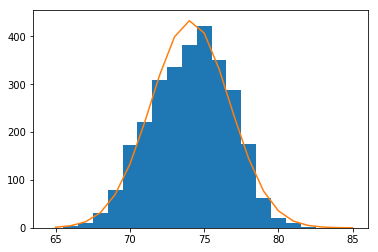The height distribution looks pretty close to normal. There's a slight overrepresentation of heights a little above the mean. But it's mostly symmetrical, has a single mode close to the mean, and has very few observations outside two or three standard deviations.

Now let's look at weight. In class I initially guessed it was "Weight (pounds)". Good thing someone checked!

In :
player_weights = [ int(p["Weight (lbs)"]) for p in active_players ]

In :
numpy.mean(player_weights)

Out:
243.4473409801877
In :
numpy.std(player_weights)

Out:
46.37809609142948
In :
## The bins argument tells the hist() function how to allocate
## data values into the bars. I chose these settings by inspecting
## different values.

pyplot.hist(player_weights, bins=numpy.linspace(150,360, 42))
pyplot.show()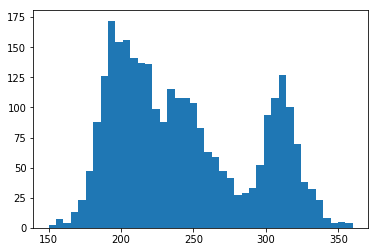That doesn't look at all normal-distribution! There are three modes (peaks). The mean is not the most common value. And a lot of values are quite far from one standard deviation away from the mean (210-280). What this looks like is at least three separate distributions added together.

Here's where some real-world knowledge comes in. Football players have quite different body types based on their role in the game. If we select only one position, say offensive tackles, we get something closer to what we expect.

In :
ot_weights = [ int(p["Weight (lbs)"]) for p in active_players if p["Position"] == "OT" ]
pyplot.hist(ot_weights, bins=numpy.linspace(150,360, 42))
pyplot.show()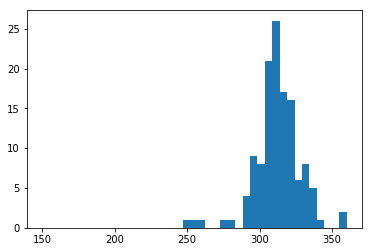In :
player_exp = []

for player in active_players:
if player["Experience"] == "Rookie":
player_exp.append(1)
else:
player_exp.append(initial_number(player["Experience"]))

print(player_exp[0:10])

[3, 1, 1, 8, 4, 8, 1, 2, 1, 6]

In :
pyplot.hist(player_exp, bins=range(1, 23))
pyplot.show()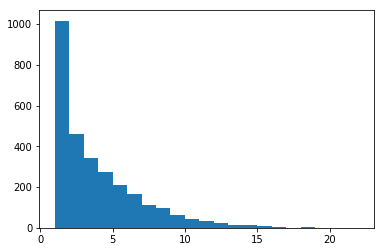In :
numpy.mean(player_exp)

Out:
3.440736878693083
In :
fake_exp = numpy.random.geometric(1/3.44, size=2700)
pyplot.hist(fake_exp, bins=range(1,23))
pyplot.show()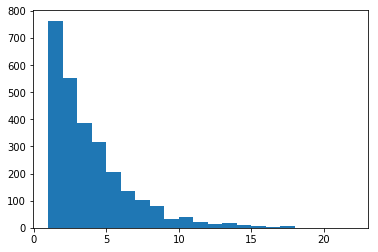In :
active_players["Birthday"]

Out:
'3/31/1992'
In :
bday_pattern = re.compile("(\d+)/(\d+)/(\d+)")
#print(dir(bday_pattern))

In :
bday_match = bday_pattern.search("3/31/1992")
#print(dir(bday_match))

In :
bday_match.group(1)

Out:
'3'
In :
bday_match.group(3)

Out:
'1992'
In :
bday_match.group(0)

Out:
'3/31/1992'
In :
bday_unmatch = bday_pattern.search("djflksjfs")
print(bday_unmatch)
if bday_unmatch:
print("dfsdf")
if bday_match:
print("match!")

None
match!

In :
player_months = []
for p in active_players:
bday_match = bday_pattern.search(p["Birthday"])
if bday_match:
player_months.append(int(bday_match.group(1)))

print(player_months[0:10])

[3, 1, 3, 4, 7, 11, 5, 1, 1, 11]

In :
pyplot.hist(player_months, bins=range(1,14), width=0.7)
pyplot.show()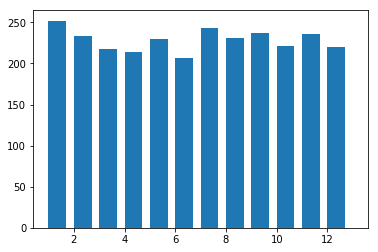In :
len(player_months)

Out:
2742
In :
len([ m for m in player_months if m <= 6 ])

Out:
1354
In :
fake_nfls = numpy.random.binomial(2742, 0.5, size=1000)
more_extreme = fake_nfls[ fake_nfls <= 1354 ]
more_extreme[0:10]

Out:
array([1345, 1329, 1335, 1341, 1345, 1353, 1310, 1348, 1352, 1342])
In :
len(more_extreme)

Out:
265
In :
mid_year = numpy.random.binomial(243 + 207, 0.5, size=1000)
more_extreme = mid_year[ mid_year <= 207 ]
print(len(more_extreme))

61